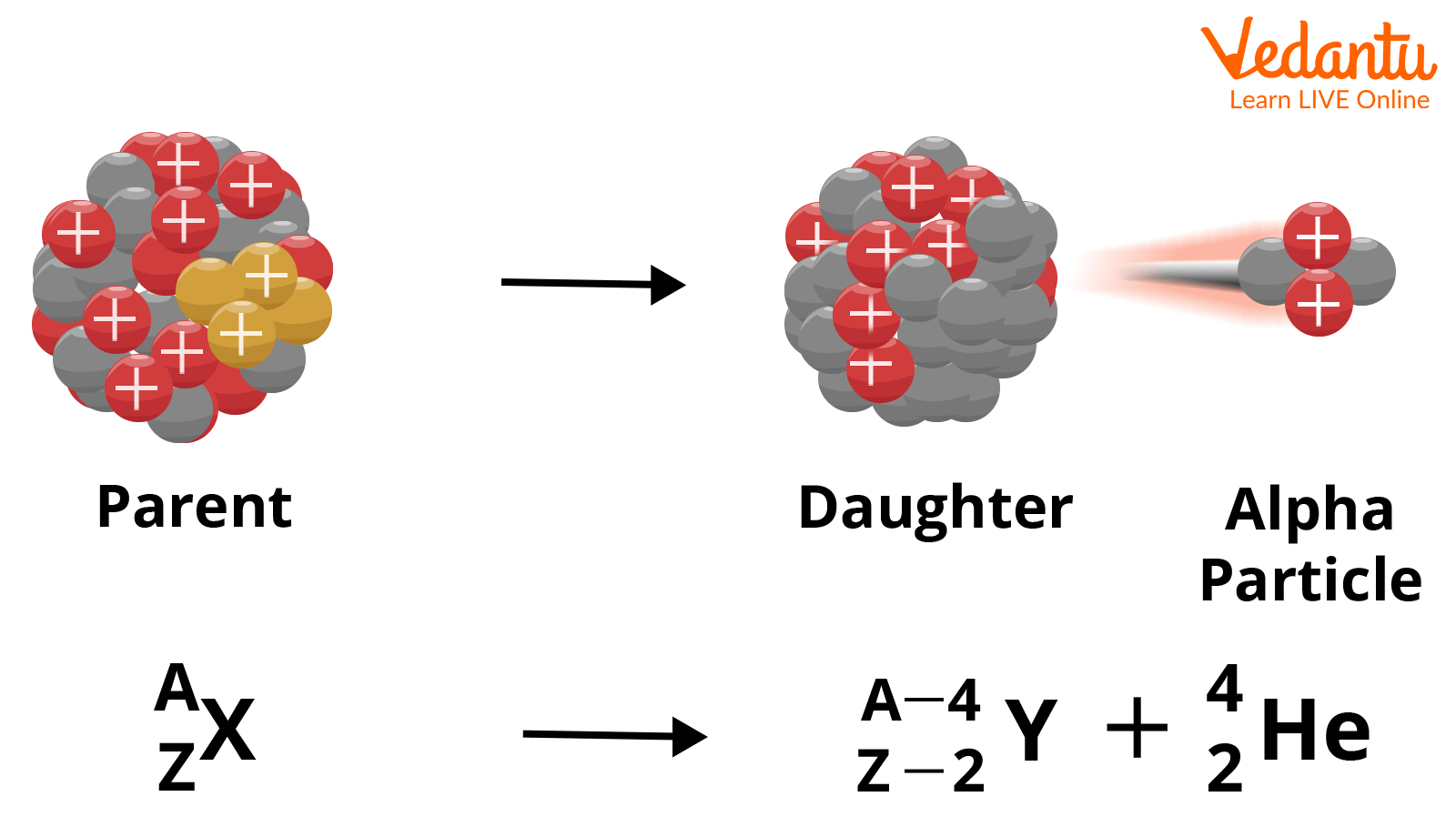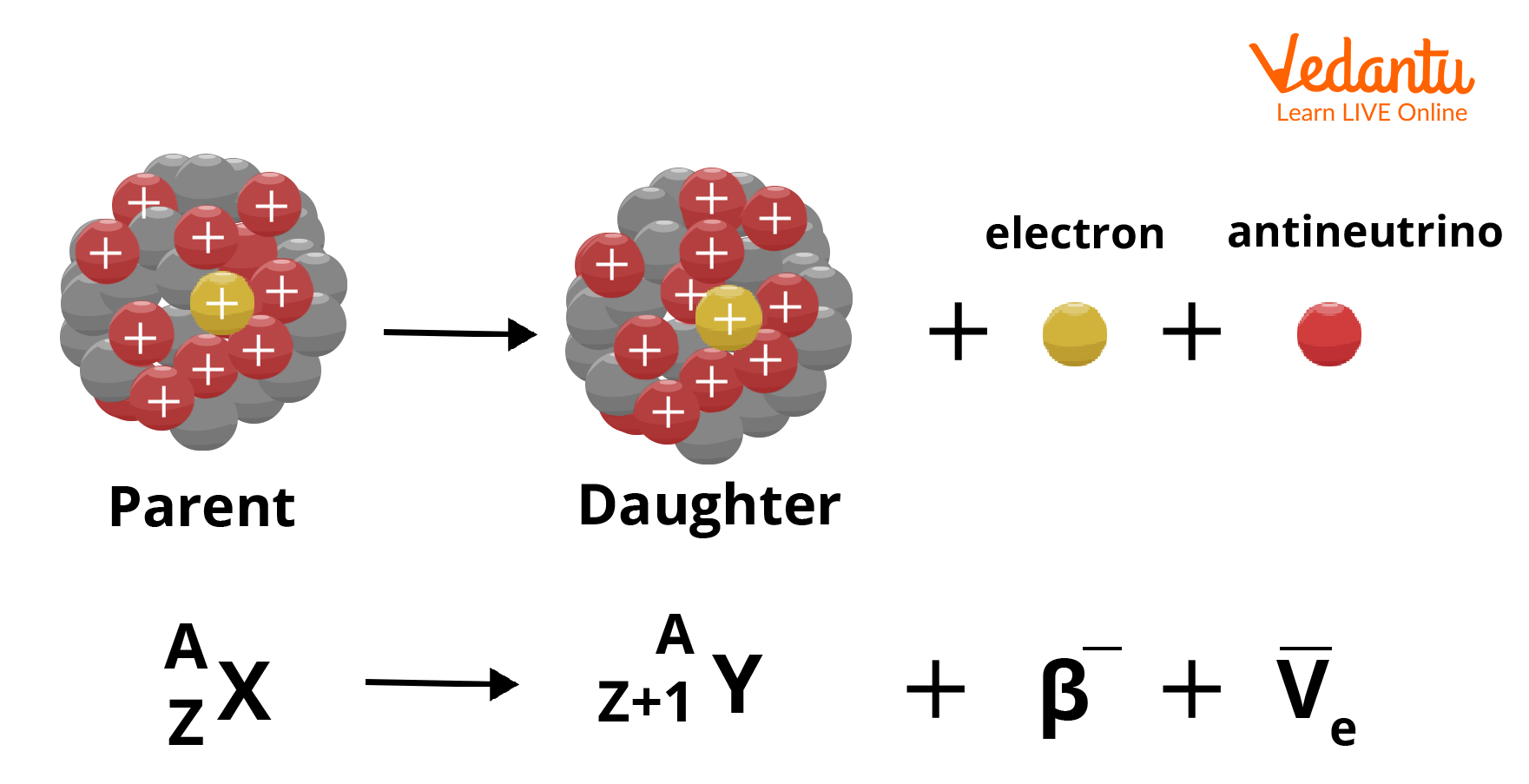Courses
Courses for Kids
Free study material
Free LIVE classes
More

# Brief Information on Alpha, Beta and Gamma Decay - JEE Important TopicLast updated date: 18th Mar 2023
Total views: 77.1k
Views today: 1.56k

Radioactive decay is a process of disintegration of the atomic nuclei present in an unstable form, and further transformed into a stable form of the atomic nuclei along with the release of high-energy particles in the form of energy. Such a decay process is recognized as a first-order process with a statistically random order, where the mass gets converted into energy. It is a type of statistical process.

In the process of this chemical reaction, million times of energy is liberated and an exothermic reaction occurs. The release of energy is in terms of energy per nucleus of a unit's electron volts (eV) and million electron volts (MeV). There are three types of radioactive decay process as given below:

1. Alpha decay process

2. Beta-decay process

3. Gamma decay process

## What are Alpha, Beta, and Gamma Decay?

An unstable nucleus in an attempt to achieve stability decays through alpha, beta and gamma decay.

### Alpha Decay:

The alpha decay process is a type of radioactive decay process, in which the fixed number of neutrons and protons change due to the unstable form of the nuclei into a stable form, through disintegration. In general terms, it can be said that the alpha decay process is a chemical reaction, where the conversion of the unstable nucleus of an atom takes place and is converted into a stable form of another atom, by releasing two protons and two neutrons in the form of exothermic energy.

Since the releasing particles are in the form of high-energy particles (like alpha particles), this process is termed as the alpha decay process. These alpha particles are positively charged helium atoms with an atomic number of 2 and mass number 4. These alpha particles originate from the alpha decay process and have a larger mass, due to which they are unable to pass through solid surfaces, but can travel for a long range in the air.

### Mechanism of Alpha Decay:

The alpha decay occurs only for huge nuclei with a mass number greater than $210$. Due to a large number of nucleons, the stability of the nucleus gets affected and an imbalance is created between the electromagnetic force and nuclear force. It is given that the strength of the nuclear force is proportional to the number of nucleons, but the repulsive electromagnetic force is proportional to the square of the nucleon’s number.Alpha Decay Mechanism

The mechanism of alpha decay maintains a balance between the strong nuclear binding forces and repulsive electromagnetic forces among the protons inside the atom, for a stable nucleus.  The general formula of alpha decay is given as:

${}_{Z}^{A}E\to {}_{Z-2}^{A-4}{{E}^{'}}+{}_{2}^{4}He$

Where,${}_{Z}^{A}E=$ Parent nucleus, ${}_{Z-2}^{A-4}{{E}^{'}}=$Daughter nucleus and ${}_{2}^{4}He=$Released alpha particles.

The example of the alpha decay process is given below:

${}_{92}^{238}U\to {}_{90}^{234}Th+{}_{2}^{4}He$

The disintegration of ${}_{92}^{238}U$ into ${}_{90}^{234}Th$ occurs through the alpha decay process. In this equation, the uranium ${}_{92}^{238}U$ undergoes a decay process in which it decomposes into the element thorium ${}_{90}^{234}Th$ with two protons and two neutrons. Since the decay process ends with the release of alpha particles $\left( {}_{2}^{4}He \right)$ with thorium, this process is an alpha decay process.

### Beta Decay:

Beta decay is the process where the disintegration of the nucleus will take place by emitting an electron or positron. This type of radioactive decay process is spontaneous in nature. It can occur in two ways:

• Negative beta $\left( {{\beta }^{-}} \right)$ decay

• Positive beta $\left( {{\beta }^{+}} \right)$ decay

In the mechanism of the beta decay process, the nucleus gets decomposed and the proton gets converted into a neutron and vice versa. In this process, if the proton is converted into a neutron, then it is a positive beta decay or beta plus decay process. If the process makes the conversion of a neutron into a proton, then it is negative beta decay or beta minus decay.

1. Negative Beta $\left( {{\beta }^{-}} \right)$ Decay:

In the beta minus decay, the neutron of the element gets decayed into a proton, electron and antineutrino. The mechanism of ${{\beta }^{-}}$ decay is shown below:

${}_{Z}^{A}E\to {}_{Z+1}^{A}C+{{e}^{-}}+\overline{v}$

The example of beta minus decay is shown below:

${}_{15}^{32}P\to {}_{16}^{32}S+{{e}^{-}}+\overline{v}$

The neutron of elements ${}_{15}^{32}P$ loses electron $\left( {{e}^{-}} \right)$ and antineutrino $\left( \overline{v} \right)$  in the given beta minus decay process.Beta Negative Decay Mechanism

2. Positive Beta $\left( {{\beta }^{+}} \right)$ Decay:

In beta plus decay, the nucleus of the element undergoes a decay process, in which a positron gets released with the neutrino in the nuclear reaction. In general terms, a proton present in the parent nucleus is converted into neutrons and emits neutrino and positron during the nuclear reaction. The general mechanism of beta plus $\left( {{\beta }^{+}} \right)$ decay is shown below:

${}_{Z}^{A}E\to {}_{Z-1}^{A}C+{{e}^{+}}+v$

The example for the beta plus decay is shown below:

${}_{11}^{22}Na\to {}_{10}^{22}Ne+{{e}^{+}}+v$

In this given process, the nucleus of the atom $\left( {}_{11}^{22}Na \right)$ undergoes a beta plus decay process and loses a positron $\left( {{e}^{+}} \right)$ with neutrino $\left( v \right)$  during this beta plus decay process.Beta Positive Decay Mechanism

### Gamma Decay:

About the atomic energy levels, a nucleus consists of different energy levels, where the basic level of energy is the ground state. The above energy level from the basic is the excited state having a higher energy than the ground state. The spacing between the ground states and the excited states of the atomic energy levels is in the form of energy of the order of eV (electron volt), while the difference of energy in nuclear energy levels is of the order of MeV (Mega electron volt).

### Mechanism of Gamma Decay:

In the gamma decay process, higher energy particles, which are termed photons, get released in the form of electromagnetic waves, due to the spontaneous decay of the nucleus to the ground state from the excited states, due to the difference in the energy levels of the nucleus at both states.

This radioactive decay process is known as gamma decay. A common example of gamma decay is successive emission of gamma rays, when $\left( {}_{27}^{60}Co \right)$ nucleus undergoes radioactive decay and gets transformed into$\left( {}_{28}^{60}Ni \right)$. This excited state nucleus reaches the ground state by the emission of two gamma rays having energies of order 1.17 MeV and 1.33 MeV.Gamma Decay Mechanism

Radioactive decay law states that the rate of probabilistic decay of the nucleus per unit of time will be constant and that constant is known as the decay constant having the symbol of ‘$\lambda$’. This constant of proportionality is different for the different nuclei of the atoms, which have a direct influence on the rate of radioactive decay. According to the law of radioactive decay, the number of nuclides at time $t$ i.e. $N(t)$ will be:

$N(t)={{N}_{0}}{{e}^{-\lambda t}}$ Here, ${{N}_{0}}=$ Initial number of nuclides

It has been found that the disintegration of the radioactive process is a type of random process, which occurred at different levels of atoms with different energy levels according to quantum theory.

## Difference Between Alpha, Beta and Gamma Decay

Let us compare them on several parameters:

 S.No. Alpha Decay Beta Decay Gamma Decay 1. In this process, a fixed number of protons and neutrons are released in the form of energy during the disintegration process. In this process, the electron and positron are emitted in the form of energy due to the disintegration of the nuclei. In this process, the electromagnetic waves in the form of photons get released due to the spontaneous decay of the nucleus due to energy difference between ground and excited states. 2. Two protons and neutrons get released in the form of alpha particles. The electron gets released in the form of beta particles. The electromagnetic waves are emitted in the form of photons. 3. The charge of the alpha particle is positive. The charge of beta particles is negative. The charge of gamma rays is neutral. 4. These particles have minimum penetration power. These particles have a higher penetration power than alpha particles. These particles have maximum penetration power in comparison with the alpha and beta particles. 5. It has maximum ionisation power. It has less ionisation power than alpha. particles, but is higher than gamma rays. It has the least ionisation power as compared to the alpha and beta particles.

## Conclusion

This article concludes with some knowledgeable aspects of alpha, beta and gamma decay.  It also explains the governing law of the radioactive decay process, which describes the emission of higher energy in the form of exothermic reactions in a spontaneous manner. This article discusses the equation of alpha, beta and gamma decay along with the examples of alpha, beta and gamma decay. Various alpha, beta and gamma decay equations are also elaborated briefly.

Every element has its specific atomic configuration, which decides the properties of the element. It was also observed that few elements have a heavier nucleus that depicts radioactivity decay through alpha, beta and gamma decay. It is imperative that the alpha particle is positively charged, a beta particle is a form of electron and the gamma particle is an electromagnetic wave.

## FAQs on Brief Information on Alpha, Beta and Gamma Decay - JEE Important Topic

1. What is the weightage of nuclear physics in the JEE exam?

The weightage of nuclear physics in the JEE exam is 5%. This section comes in the category of low weightage for the JEE exam. The concepts involved are nuclear fusion and fission, radioactive decay process and its law, alpha decay process, gamma decay process, beta decay process and half time relating numerical. As this section holds less weightage in the JEE exam, it is advised not to waste extra time for remembering the concepts regarding nuclear physics.

2. What is the charge of the particles emitted from the alpha, beta and gamma decay?

The charge for the emitted particles in the respective decay process is given below:

• The charge for the particles emitted from the alpha decay process is positive. During this alpha decay process, the alpha particles can only be formed when the two protons and two neutrons are bound together.

• The charge for the beta particles emitted from the decay process is negative. During this beta decay process, the negatively charged particles which were known as electrons, emit with very high speed and are acknowledged as beta particles.

• The charge for the gamma particles emitted from the gamma decay process is neutral.

3. What is the meaning of disintegration in the radioactive process?

The meaning of disintegration in the radioactive decay process is the decomposition of unstable nuclei into their stable form after the liberation of energy. The disintegration leads to the breaking of the unstable parent nucleus and gets converted into the stable form of the nucleus, along with the release of radiation in the form of alpha, beta and gamma particles during the radioactive process.

In the alpha decay process, the alpha particles get emitted. In the beta decay process, the beta particles get emitted and gamma particles get emitted in the gamma decay process. One of the common example of disintegration process is shown below:

${ }_{92}^{238} U \rightarrow~~{ }_{90}^{234}Th~+~{ }_{2}^{4}He$